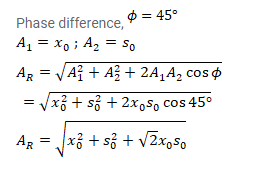# A particle is subjected to two simple harmonic motions,

Question:

A particle is subjected to two simple harmonic motions, one along the X-axis and the other on a line making an angle of $45^{\circ}$ with the $X$-axis. The two motions are given by $x=x_{0} \sin \omega t$ and $s=s_{0} \sin \omega t$

Find the amplitude of the resultant motion.

Solution: